# Curvature is symmetric in the pairs of first and last two variables

Jump to: navigation, search

## Statement

Suppose$M$ is a differential manifold and$g$ is a Riemannian metric or pseudo-Riemannian metric on$M$. Suppose$\nabla$ is the Levi-Civita connection on$M$ and$R$ is the Riemann curvature tensor for$\nabla$. (In other words,$R$ is the Riemann curvature tensor of the Levi-Civita connection).

We can view$R$ as a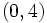$(0,4)$-tensor as follows: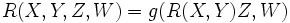$\! R(X,Y,Z,W) = g(R(X,Y)Z,W)$.

Then, we have: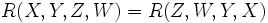$R(X,Y,Z,W) = R(Z,W,Y,X)$.

Note that since curvature is antisymmetric in first two variables and curvature is antisymmetric in last two variables, this essentially shows that$R$ is a symmetric bilinear form on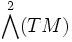$\bigwedge^2(TM)$.

## Facts used

1. Curvature is antisymmetric in first two variables
2. Curvature is antisymmetric in last two variables
3. First Bianchi identity: This states that if$\nabla$ is a torsion-free linear connection, and$R$ is its Riemann curvature tensor, then: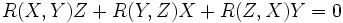$\! R(X,Y)Z + R(Y,Z)X + R(Z,X)Y = 0$.

For the corresponding$(0,4)$-tensor, we have:$\! R(X,Y,Z,W) + R(Y,Z,X,W) + R(Z,X,Y,W) = 0$.

## Proof

Applying fact (3), we get:$\! R(X,Y,Z,W) + R(Y,Z,X,W) + R(Z,X,Y,W) = 0 \qquad (1)$

Similar statements, permuting the variables, are: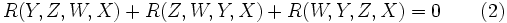$\! R(Y,Z,W,X) + R(Z,W,Y,X) + R(W,Y,Z,X) = 0 \qquad (2)$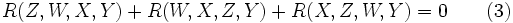$\! R(Z,W,X,Y) + R(W,X,Z,Y) + R(X,Z,W,Y) = 0 \qquad (3)$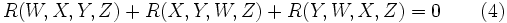$\! R(W,X,Y,Z) + R(X,Y,W,Z) + R(Y,W,X,Z) = 0 \qquad (4)$

Consider (1) + (2) - (3) - (4) and uses facts (1) and (2). We get: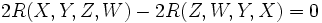$\! 2R(X,Y,Z,W) - 2R(Z,W,Y,X) = 0$

This completes the proof.